# Dstar Lite: An Optimal Algorithm for Robotics Pathfinding

0
13263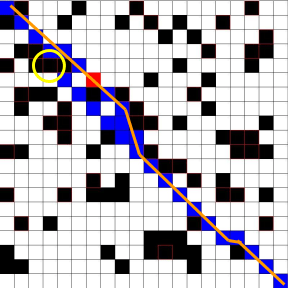Editor’s note: This manuscript is being posted as a preprint. It is currently in the process of peer review, and we are awaiting author revisions. In the meantime, a copy of the submitted manuscript is posted here for advance consideration.

Jeffrey F. Zhang (student)1, Tony. Dear (mentor)2

## Abstract

Dstar Lite is a complex pathfinding algorithm with practical applications in many fields such as robotics and game design. This algorithm is capable of solving the shortest path problem and also incorporates additional functionality such as path replanning and path correction. Through the use of a computer simulation and analysis of the algorithm we tested the capabilities and processing speed of the algorithm to see if it could be a viable tool in a High School robotics competition setting. Ultimately, we found that Dstar Lite works more efficiently than similar algorithms as it provides a fast and reliable path that leads from the start to the end. We recommend that robotics teams implement this algorithm into their own robot code and use it for autonomous movement.

## Keywords

Robotics, Control Theory, Path planning, Astar, Dstar-Lite.

## Introduction

Dstar Lite is a pathfinding algorithm that allows the programmer to find the optimal path between a starting point and an ending point in a known or partially known environment. This algorithm was designed to be run on a graph data structure consisting of nodes connected by edges. The nodes that point towards the current position on which the pathfinder is located are called the predecessor nodes while the nodes the current node points towards are called its successors.Figure 1. Describe what a successor and a predecessor is for a given node in a graph

Dstar Lite works by keeping track of two scores for each node on a given graph. The first score is the G score. This value keeps track of the cost of arriving at a position on the graph. It can be thought of as the shortest path that connects the starting point to the current point. For the starting point, the G score is zero because the pathfinder is already there. The RHS score, on the other hand, is a more useful estimate. This score acts as a one-step look ahead and, in the algorithm, it is used to find the next optimal position for the pathfinder to travel to. If the next position is an obstacle, the connection costs would be set to infinity because the pathfinder can never reach that point. As a result, the RHS score is also infinity. Using these two scores, the algorithm propagates a path from the ending point to the start point eventually finding the optimal path from start to finish.

``````Terminology:
S = current node
S’ = predecessor node
C (s’, s) = edge cost for s’ ? s
G(s) = current cost to arrive at that node
G(s) = G(s’) + C (S’, S)
RHS(s) = min value((G(S’) + C (S’, S))
``````

The G score and RHS score act as the foundation for Dstar Lite path planning and replanning purposes. In the event the Dstar Lite algorithm encounters unforeseen obstacles and is forced to replan the path, the RHS score is simply recalculated with the new connectivity cost for all affected nodes. A more detailed explanation can be found in Koenig and Likhachev’s paper on the Dstar Lite algorithm.

Dstar Lite example run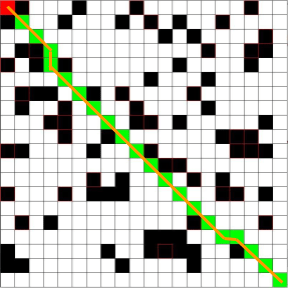Figure 2 – Robot creates initial optimal path with all known information. Green/Blue Tile = PathRed Tile = PathfinderBlack Tile = ObstaclesYellow Circle = appearing obstacle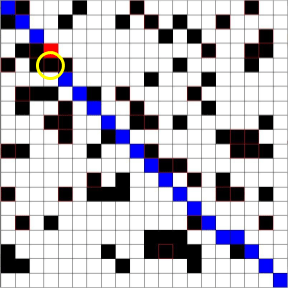Figure 3 – While moving down the path the robot encounters an obstacle Green/Blue Tile = PathRed Tile = PathfinderBlack Tile = ObstaclesYellow Circle = appearing obstacleFigure 4 – Robot readjust path using Dstar Lite and finds the next optimal path Green/Blue Tile = PathRed Tile = PathfinderBlack Tile = ObstaclesYellow Circle = appearing obstacle

This algorithm has many practical uses in industry. Most notably Dstar Lite is used in the telecommunication industry when cell signals have to find the optimal path from a starting spot to the destination by relaying the signal from a network of cell towers. In addition, this algorithm is often best for path planning in the field of robotics. Dstar Lite allows for quick replanting in a dynamic environment. This function is useful for a robot because in the real world the environment is ever changing and the robot needs to be able to avoid obstacles and quickly replan its path.

Before Dstar Lite was developed in 2002 other algorithms such as Astar and Dijkstra’s algorithm dominated the area of path finding. Dstar Lite differs from these path optimization algorithms in two main ways. First, Dijkstra and Astar require the user to have complete knowledge of the environment as they do not provide built in replanning functionality. This difference means that if the robot encounters something unknown, the Astar and Dijkstra’s algorithms would need to completely rerun so a new path would be created. Dstar Lite, on the other hand, quickly recalculates only the affected areas, thus significantly reducing the necessary processing time. When a node is detected to be an obstacle, the cost of arriving there becomes infinity and so the RHS score is updated to infinity. Secondly, the Astar and Dijkstra’s algorithms start by generating a path from the start to finish. Dstar Lite, on the other hand, generates the path from the goal to the start. This difference is useful for replanning because when a path needs to be regenerated fewer changes are necessary when traversing backwards.

In our paper we will discuss how Dstar Lite is an effective algorithm for the field of robotics as well as for use in high school robotics competitions. We believe that the algorithm’s capabilities can be used for autonomous movements and assist with navigation.

## Results and Discussion

The first test we conducted with our simulation investigated how run time changed as the size of the grid increased. In our test we used grid length and widths starting at 10 by 10 and then slowly increased the size until it reached 500 by 500. Through this test we observed that as the grid increased in dimensions the run time drastically grew. This is expected as when the length and width of the grid increases, the number of tiles (equal to the length times width) grows quadratically. As Dstar Lite traverses through the grid, it has to check the 8 tiles that are directly neighboring it. When there are more coordinates in the total grid, Dstar Lite checks more tiles overall and as a result the run time drastically increases. This observation is shown in the chart and the graph below.

Table 1 – Grid Size versus Run Time

Next, we tested how the number of obstacles on the field affected the run time. This result was more surprising because we saw that as more obstacles were placed on the grid the run time actually decreased. We believe that this occurred because having more obstacles on the grid reduces how many points the path finding algorithm has to check. In our test we started with ten percent of the field filled and slowly increased the percentage of the grid filled with obstacles to seventy percent. We noticed that the run time consistently decreased as more obstacles were added. This finding was surprising because we thought that more obstacles would mean the path had to be more curved and irregular. Instead it appears that when there is an abundance of obstacles, the number of points Dstar Lite checks drastically reduces.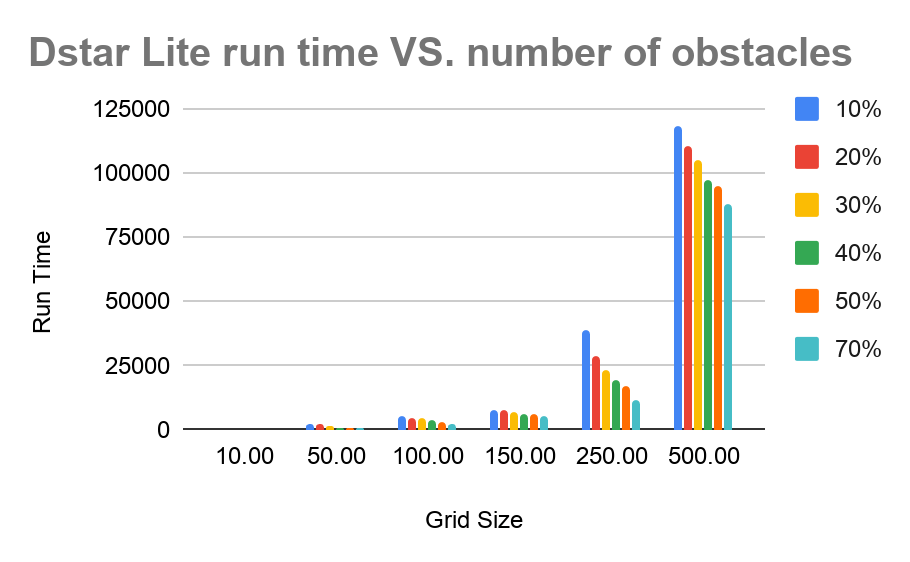Figure 6 – Run Time of Dstar Lite algorithm for different grid sizes and different amount of obstacles

Lastly, we compared the run times of Dstar Lite and Astar. When obstacles were randomly introduced while the algorithm traversed from start to finish, we saw that Dstar Lite is faster than the Astar algorithm. Since Astar did not support replanning, the algorithm has to be rerun from the current point to the end point meaning it has to do redundant work. Dstar Lite on the other hand was more efficient at this process and uses past information to replan faster.

Table 2 – Dstar Lite vs Astar run time

In addition to experiments regarding obstacles and field size we also tested to see if this algorithm was capable of path correction. Path correction is needed when a robot follows a path but due to friction and other mechanical and physical errors, it deviates from the planned path and ends up somewhere else. We tested to see if the Dstar Lite algorithm can be used to replan the path and lead the robot back in the correct direction. We tested this by adding random movement errors as the simulated robot traversed the grid. Every time the robot moves from one tile to the next in the path there is a small probability that the robot would deviate from the correct position and end up at another neighboring tile instead. When this occurred, we replanned the path using Dstar Lite and continued following the newly generated path. During our experiment we saw that this behavior is identical to what Dstar Lite would do when its path is blocked by an obstacle. Interestingly, when this occurred, we saw that the algorithm either generated a completely new path to end or it would try to fix itself and lead the robot back to the original path.

## Discussion

Dstar Lite is an algorithm that is widely used in the field of robotics. This path planning algorithm can be implemented with odometry and create accurate motion profiling functionality for a real robot. In addition, this algorithm can be used in conjunction with a sensor array to dynamically avoid obstacles while the robot is moving. We think that this algorithm can have an impact in competition robotics because this software solves many issues such as obstacle avoidance and accurate movement control. Currently many of the challenges that robotics teams face when creating autonomous code can be solved by adapting the Dstar Lite algorithm into their software.

In our experiment, we observed that on an open grid with few obstacles Dstar Lite is less efficient than on a crowded grid with many obstacles. We believe that this occurred because in our adaptation of Dstar Lite, when a tile is occupied by a barrier, Dstar Lite overlooks the spot and moves on. This would mean that if a grid had many barriers, the pathfinder would skip more tiles and the processing time for the algorithm would decrease. This effect appears to be beneficial as it can be used to decrease necessary processing power and allow for quicker path generation.

We also found that there was generally a lot of variability for runtimes during each test. This was most likely caused by the background process running on the test computer as well as the variability of where obstacles were placed. In order to minimize these effects we ran multiple trials for each condition and then averaged the run times to create a more accurate value. In addition, whenever random barriers were placed on the grid there is a small chance that they created a barrier so there was no possible path between start and finish. If this occurred, we regenerated all of the obstacles and then redid the trial.

Lastly, since we are using a grid with discrete tiles to keep track of robot position, Dstar Lite is unable to generate the absolute optimal path. The absolute optimal path between two points is generally a straight line from start to finish that perfectly wraps around obstacles. A grid like this which has eight possible neighboring spots to traverse would limit the algorithm from generating a true perfect path like the one shown below.Figure 8 – Dstar light optimal path vs true shortest path. Green: generated path. Orange: true shortest path.

This error could be solved using a line of sight algorithm. Instead of only considering neighboring tiles to be a successor, a line of sight algorithm would consider any tile with direct line of sight to be a successor of the current tile. This would allow the path generated to be straighter and more fined tuned. Similarly, this problem can also be improved by increasing the resolution of the graph. In this case we would simply make the grid larger with more rows and columns. This would increase the accuracy of the path but the runtime would also increase. Both of these methods would improve the path but it would also mean that more processing power and runtime is needed.

## Conclusion

In this paper, we show the efficacy of Dstar Lite as a planning algorithm. We compared Dstar Lite against Astar and discovered that the former algorithm is substantially faster at replanning. We believe that this algorithm is extremely useful in the field of robotics and can easily be implemented in a high school robotics competition setting. Current high school competitions often use game fields that are rectangular grids with known and unknown obstacles placed on top. Dstar Lite would be capable of avoiding these obstacles and allowing the robot to quickly find the shortest path. Not only is this algorithm extremely efficient at creating paths, it is faster than similar algorithms such as Astar in path replanning and obstacle avoidance. We recommend that robotics implement this algorithm into their autonomous code.

## Methods

While Dstar Lite was originally designed to be run on a graph with specific nodes, for our experiment, we decided to use a square grid containing N rows and N columns. We used this setup because a grid can easily correspond to the Cartesian coordinate system and can be represented as a two-dimensional array in code. This means that the (0,0) position, or the origin on the grid, corresponds to the (0,0) position on the cartesian plane (note that in computer rendering, the (0,0) point is defined as the top left corner and the following pictures show the origin there). In addition, a grid structure allows for ease of calculations. In a traditional graph, the connection cost between each node needs to be implicitly defined and stored. For a graph with many nodes and connections this would quickly add up and consume memory. In our grid the cost of each connection can be automatically defined as the distance between each point. This allows us to calculate the connection cost using the distance formula. Similarly, the predecessors and successors of a graph need to be implicitly defined. Once again, a grid optimizes this process. The predecessors and successors of the current node on a grid are simply the eight nodes that neighbor it.

Inside the grid the robot was initialized at the coordinate (0,0) (top left of the field) and the end goal was set to be at (N-1, N-1) (the bottom right of the grid). This coordinate is defined as (N-1, N-1) because we used 2D arrays as our data structure and arrays start at zero. These two points were chosen to ensure that the path would be as long as possible and so the pathfinder would be required to face more obstacles during the path creation process.

Lastly a different number of obstacles were randomly placed on the field before each run. These obstacles were placed to simulate blocks and barriers which would prevent access to the goal. In addition, while the robot moves from the starting point to the end point, obstacles have a ten percent chance of randomly appearing in front of the robot, blocking its path, and forcing the algorithm to readjust the path.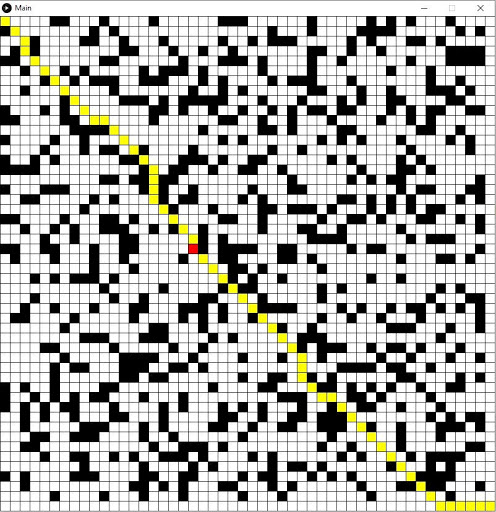Figure 10 – Example of generated environment.Red Square: RobotBlack Square: ObstacleYellow Square: Generated PathWhite Square: Empty Spot

Through the use of our simulation environment we experimented to determine if this algorithm is efficient enough to be used in a competition robotics setting. In many high school robotics competitions, competitors are required to build autonomously driven robots to navigate complex environments to complete a certain task. We tested to see how well this algorithm can be used in such a setting.

Throughout our experiment we looked at three different factors: how changes in the size of the field affected the algorithm’s run time, how the number of obstacles affected the run time, and how the Dstar Lite algorithm compared to the Astar algorithm in regenerating an optimal path. In total we ran 84 trials using Dstar Lite and then ran 42 trials using Astar. For each different condition we ran six trials and then averaged the values.

## Acknowledgements

We would like to thank Professor Dear and Christopher Brown for providing guidance and help during the process. Professor Dear was crucial in the experimental process and helped introduce us design the simulations as well as in debugging. In addition, we would like to acknowledge Christopher as he helped us work on the final report and helped us look at the project from a different perspective.

## References

(1) Choset, H. https://www.cs.cmu.edu/~motionplanning/lecture/AppH-astar-dstar_howie.pdf (accessed Jul 18, 2020).

(2) Koenig, S.; Likhachev, M. D* Lite. http://idm-lab.org/bib/abstracts/papers/aaai02b.pdf (accessed Jul 18, 2020).

(3) Buniyamin, N.; Sariff, N. An Overview of Autonomous Mobile Robot Path Planning Algorithms – IEEE Conference Publication. https://ieeexplore.ieee.org/abstract/document/4339335/authors#authors (accessed Jul 18, 2020).

(4) Introduction to A*. http://theory.stanford.edu/~amitp/GameProgramming/AStarComparison.html (accessed Jul 19, 2020).

(5) Konakalla, S. A Star Algorithm. http://cs.indstate.edu/~skonakalla/paper.pdf (accessed Jul 19, 2020).

(6) Language Reference (API) \ Processing 3+. https://processing.org/reference/ (accessed Jul 19, 2020).

(7) Kang, H.; Lee, B.; Kim, K. Path Planning Algorithm Using the Particle Swarm Optimization and the Improved Dijkstra Algorithm – IEEE Conference Publication. https://ieeexplore.ieee.org/abstract/document/4756927 (accessed Jul 19, 2020).